### IMO Shortlist 1971 problem 3

Kvaliteta:
Avg: 0,0
Težina:
Avg: 0,0
Knowing that the system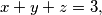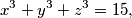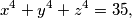has a real solution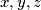$x, y, z$ for which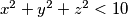$x^2 + y^2 + z^2 < 10$, find the value of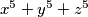$x^5 + y^5 + z^5$ for that solution.
Izvor: Međunarodna matematička olimpijada, shortlist 1971